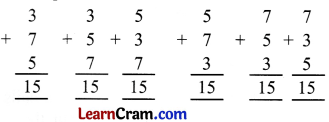# DAV Class 4 Maths Chapter 2 Worksheet 2 Solutions

The DAV Class 4 Maths Book Solutions and DAV Class 4 Maths Chapter 2 Worksheet 2 Solutions of Addition and Subtraction offer comprehensive answers to textbook questions.

## DAV Class 4 Maths Ch 2 WS 2 Solutions

Question 1.
Fill in the blanks.
(a) 75,361 + 2,135 = 2,135 + __________
75,361

(b) 45,793 + 15,911 = __________ + 45,793
15,911

(c) 92,501 + 123 + 111 = 111 + 92,501 + __________
123

(d) 21,511 + 222 + 11,333 + __________ + 222
11,333(e) 76 + __________ + 92 = __________ + 92 + 15
15; 76

(f) __________ + 615 + 62 = 617 + __________ + 615
617; 62

(g) 75,312 + 0 = __________
75,312

(h) 0 + 9,21,216 = __________
9,21,216

(i) 5,79 ,301 + 0 = 0 + __________
5,79,301

(j) 0 + 2,571 = 2,571 + __________
0

(k) 723 + __________ = 723
0

### DAV Class 4 Maths Chapter 2 Worksheet 2 Notes